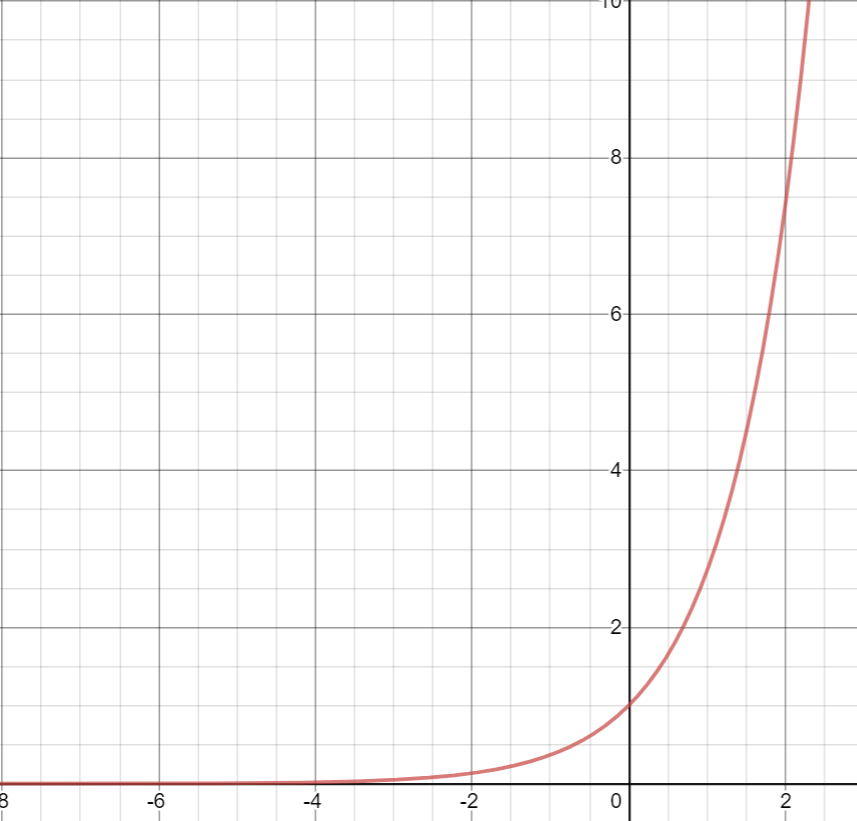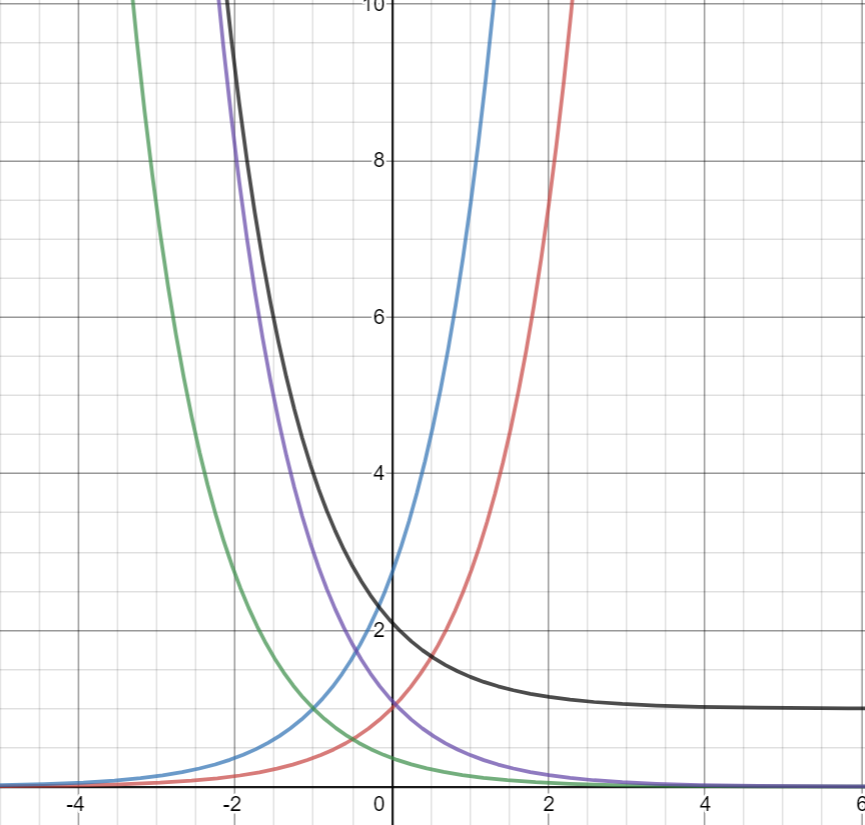# Part 7: Exponentials | Differentiating Exponentials and Solving EquationsGet confused when differentiating exponentials? This article will explain how to differentiate and solve equations involving exponentials.

## Year 11 Advanced Mathematics: Exponentials

In this article, we will discuss how to differentiate exponentials, and apply chain rule to solve more complex exponentials.

Mastering this concept will give you a better understanding of the rate at which viruses spread and a better understanding of whether Australia is successfully combatting COVID-19!

## NESA Syllabus Outcomes for Exponentials

NESA requires students to demonstrate proficiency in the following Syllabus outcomes:

E1.3 The exponential function and natural logarithms

• Establish and use the formula $$\frac{d(e^x)}{dx}= e^x$$(ACMMM100)
• Using technology, sketch and explore the gradient function of exponential functions and determine that there is a unique number $$𝑒 \approx 2.71828182845$$, for which $$\frac{d(e^x)}{dx}= e^x$$ where $$𝑒$$ is called Euler’s number
• Apply the differentiation rules to functions involving the exponential function, $$𝑓(𝑥) = ke^{ax}$$, where $$𝑘$$ and $$𝑎$$ are constants

E1.4 Graphs and applications of exponential and logarithmic functions

• Establish and use the algebraic properties of exponential functions to simplify and solve problems (ACMMM064)
• Solve problems involving exponential functions in a variety of practical and abstract contexts, using technology, and algebraically in simple cases (ACMMM067) AAM

## Assumed Knowledge

Students should be familiar with index laws.

Students should also be familiar with the concept of differentiation, and how to apply the product, quotient and chain rules.

Students can refresh their knowledge of differentiation in our Year 11 Guide Article: Calculus.

## Exponentials

### Differentiation of Exponentials

In calculus, when dealing with exponential functions, a common base to use is $$𝑒$$ (Euler’s number), where $$𝑒 \approx 2.71828$$. This function $$e^x$$ is called the exponential function.

The exponential function $$e^x$$ is quite special as the derivative of the exponential function is equal to the function itself.
$$\frac{d}{dx} (e^x )= e^x$$

By applying chain rule, other standard forms for differentiation include:

\begin{align*} \frac{d}{dx}e^{ax+b} = ae^{ax+b} \\
\frac{d}{dx}(e^u ) = e^u \frac{du}{dx} \end{align*}

### Example

Find the gradient of the tangent of $$y=2e^{x^2+x}$$

### Solution

This question follows the form, $$\frac{d}{dx}e^u=e^u \frac{du}{dx}$$ where $$u=x^2+x$$.
$$\frac{du}{dx}=2x+1$$, using our basic differentiation knowledge.
Hence $$y’=2(2x+1)e^{x^2+x}$$

### Derivatives of exponentials with different bases:

To derive $$a^x$$, we must use $$\frac{d}{dx}e^x= e^x$$.
$$a^x= e^{ln⁡(a^x}$$ (exponential and logarithms are inverse functions)
$$=e^{xln(a)}$$ (property of logarithm)
Therefore,

$$\frac{d}{dx}a^x= ln⁡(a)e^{xln(a)}=ln⁡(a)a^x$$

### Exponential Growth and Decay

An application of exponentials are finding the rate at which a quantity changes at a particular time. It is best to examine this through an example.

### Example

A population $$P$$ is growing at a rate which is given by the formula $$P=1000e^{0.2t}$$, where $$t$$ is the number of days.
(a) Find the rate at which the population is changing and show that $$\frac{dP}{dt}=200e^{0.2t}$$
(b) Find the average rate of change over the first $$5$$ days.
(c) When is the population increasing by $$1000$$ per day?

### Solution

(a) The rate the population is changing is the change of population over time, and hence equals to $$\frac{dP}{dt}$$.

$$\frac{dP}{dt}=1000 \times 0.2e^{0.2t}=200e^{0.2t}$$

(b)

At $$t=0, P_0=1000$$

At $$t= 5, P_5=1000e$$

\begin{align*}
Hence \ average \ rate = \frac{P_5 – P_0}{t_5-t_0} \\
\cong \frac{1718.28}{5} \\
\cong 344 people \ a \ day \\
\end{align*}

(c)

\begin{align*}
\frac{dP}{dt}=1000 \\
200e^{0.2t}=1000 \\
e^{\frac{t}{5}}=5 \\
\frac{t}{5}=ln⁡(5) \\
t=5ln⁡(5) \\
\cong 8 \ days
\end{align*}

### Graphs of exponentials

A normal exponential $$y=e^x$$ graph is shown below, and hence $$lim_{x→-\infty}⁡e^x=0$$ and there is a horizontal asymptote at $$y=0$$.We can use the usual transformations to change the exponential graphs.

## Want exponential improvement in your Year 11 Maths results?

Matrix+ online Year 11 Maths Avdanced courses are the expert guided solution to your Maths problems. Learn more.## Concept Check Questions

1. Find the derivative of $$3e^{2x+1}$$

2. Find the equation of the tangent at $$f(x)=xe^{2x+7}$$ at $$x=1$$

3. A radioactive sample with mass $$M$$ decays at such a rate that $$M=M_0 e^{-0.2t}$$, where $$M_0$$ is the original mass and $$t$$ is the time in years.
(a) How long until half the sample is gone?
(b) Find the rate at which the sample is decaying and prove that $$\frac{dM}{dt}=-0.2 \times M$$
(c) When will the rate of decay be $$2%$$ of the original mass $$M_0$$ per year?

4. Graph $$y=3e^{-(x+1)}+1$$

## Concept Check Solutions

1. Since $$\frac{d}{dx}e^{ax+b}= ae^{ax+b}$$, the derivative of $$e^{2x+1}$$ is $$2e^{2x+1}$$, then the derivative of $$3e^{2x+1}$$, is $$3\times 2e^{2x+1}= 6e^{2x+1}$$

2. We can use the product rule to solve this where $$u=x$$ and $$v= e^{2x+7}$$.
$$u’=1$$ and $$v’= 2e^{2x+7}$$
Using the product rule, $$f'(x)=2xe^{2x+7}+e^{2x+7}$$
Hence, the gradient at $$x=1$$ is $$f’ (1)=2e^9+e^9=3e^9$$
To find an exact point that this tangent goes through, $$f(1)=e^9$$. Hence, the tangent has a gradient of $$3e^9$$ and goes through the point $$(1,e^9)$$.
By substituting this into the point-gradient form of a line, and rearranging, the solution is $$y=3e^9 \times – 2e^9$$.

3.

(a) Since $$M_0$$ is the original mass, then $$\frac{1}{2} M_0$$ is half the sample.

\begin{align*} \frac{1}{2} M_0=M_0 e^{-0.2t} \\
\frac{1}{2}=e^{-0.2t} \\
-0.2t=ln⁡(\frac{1}{2} \\
t=5ln⁡(2) \approx 3.5 \ years \end{align*}

(b) $$\frac{dM}{dt}=-0.2 \times M_0 e^{-0.2t}=-0.2M$$

(c) When $$\frac{dM}{dt}=-\frac{1}{50}M_0$$,
\begin{align*} -\frac{1}{50}M_0=-0.2 \times M_0 e^{-0.2t} \\
\frac{1}{10}=e^{-0.2t} \\
t=5 ln⁡(10) \approx 11.5 \ years \end{align*}

4. We can graph this using multiple transformations:
Shift the graph $$y=e^x$$ graph to the left by $$1$$ unit to get $$y=e^{x+1}$$
Then reflect on the $$y$$ – axis, to get $$y=e^{-(x+1)}$$
Apply a vertical stretch by $$3$$, to get $$y=3e^{-(x+1)}$$
Shift the graph up $$1$$ unit, to get $$y=3e^{-(x+1)}+1$$## Want to check your progress with Exponentials?© Matrix Education and www.matrix.edu.au, 2021. Unauthorised use and/or duplication of this material without express and written permission from this site’s author and/or owner is strictly prohibited. Excerpts and links may be used, provided that full and clear credit is given to Matrix Education and www.matrix.edu.au with appropriate and specific direction to the original content.